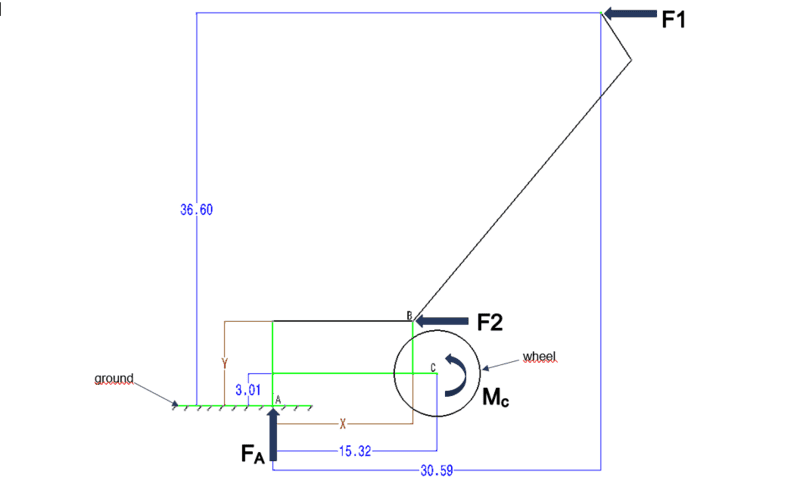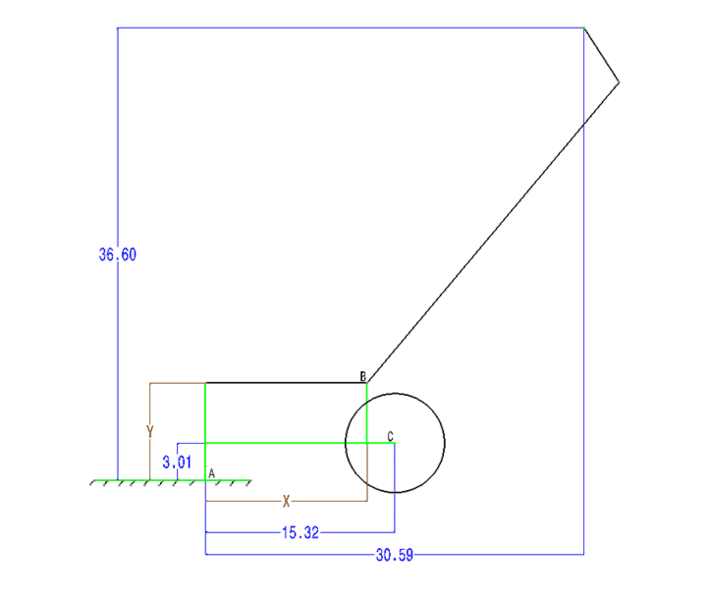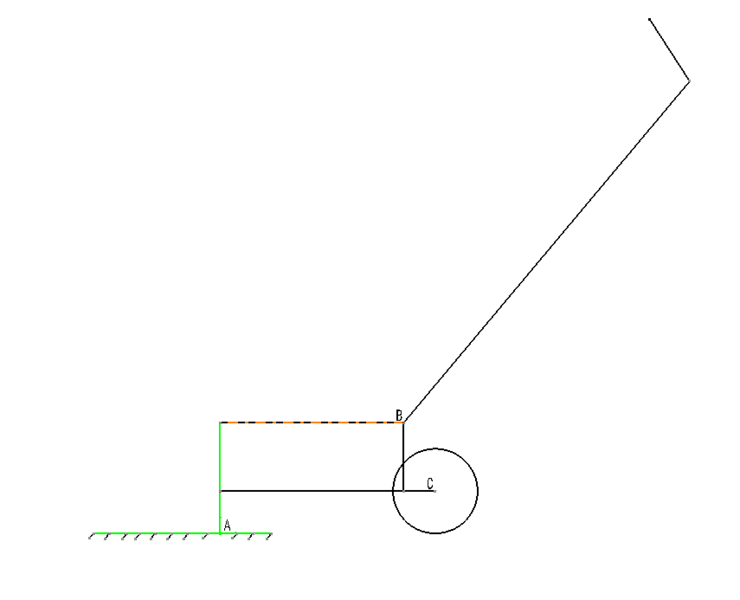# Determine the vertical reaction force

Moved from a technical forum, so homework template missing
Forgive my current brain fart, but just posting here for some confirmation of my thoughts.

I have a cart with a similar setup to a rear wheel wheelbarrow. Assuming static conditions, I am trying to determine the vertical reaction force (FA) from the ground when applying a horizontal force to the handle (F1). There is a moment Mc about the wheel (pivot point / fulcrum) and the dimensions are provided.

Per my assumptions is an getting the following results:

Value Units
Force F1 = 50 pounds
Distance Y = 7.872 inch
Distance X = 13.065 inch

Moment due to F1: M = F*d1 = 50*( 36.6-3.01) = 1679.5 inch-pound
Force: FA = M/d2 = 1679.5/(15.32) = 109.6279 poundIs the input force F1 the same as F2 despite being applied after different locations.
Am I over simplifying this or have I correctly remember what my professors taught me years ago?Thanks in advance for anyone thoughts and comments.,

## Answers and Replies

kuruman
Science Advisor
Homework Helper
Gold Member
I cannot understand what your cart looks like from what you are showing in the figure. The bottom of the wheel appears to be below ground level. Regardless of that, you provide a value for F1, but you don't seem to include the weight of the cart in your calculation. Shouldn't that be part of the action that causes the reaction from the ground?

wheel (radius 3.01) is is not drawn to scale.
I am investigating a very simplified scenario here, looking only at external forces applied to the cart. (ignore friction, cart weight, etc.)

clearer drawing of cart... the green line on the front of the cart is representing a skid plate.#### Attachments

It appears to be a question where the problem is about torque.

kuruman
Science Advisor
Homework Helper
Gold Member
That's much better, thank you. So you want to know the force FA that the ground exerts on the skid plate when you push forward with force F1 horizontally at the end of handle. Under the simplifying assumption that the cart is weightless, you calculated the reaction force by saying that the sum of the torques about the axle C is zero. It looks like you did everything right.

So, at point A, the system is fixed. The forces both normal and tangent to the ground are whatever they need to be to maintain it as static.

Here is the first problem I run into. The system is under constrained.

The radius for F1 is from point A. "Skid plate" doesn't scream fixed from rotation. Something must offset rotation about point A

kuruman
Science Advisor
Homework Helper
Gold Member
Something must offset rotation about point A
You are correct. The "something" would the weight of the cart. This calculation provides the reaction force in the limit that the cart is massless as stipulated by OP. At least that's how I understood OP's question.

So, then, the problem assumption is that point c is fixed in space and free to rotate. (Not much of a cart as it can't roll.) Then we get the equivalence of torque at c.

The problem I'm having is I'm not seeing F1 = F2, in general, just perhaps give the specific values of the problem. That requires doing some sine angle functions to get the components. Yuck

kuruman
Science Advisor
Homework Helper
Gold Member
The whole problem as stated is oversimplified because there is only one horizontal force and one vertical force. There is no way way that the sum of all the forces will be zero. One can think of friction at A providing a horizontal force, but the weight of the cart is still needed to balance the vertical component of the reaction force else you cannot have equilibrium.

i understand i have severely over simplified the problem at hand.

yes point C is fixed in space and free to rotate (since i am analyzing this "cart" in a static state to keep things simple)

Given the simplified scenario i have presented I am just trying to figure out the resultant vertical force at A when the horizontal force is F1 is applied.

There is no F2 force acting on the system. I am going off the assumption that if i were to apply F1 at the end of the handle, would the same amount of force be applied the complete length of the handle (which ends at point B)?? this bit of info is arbitrary to the presented situation.

I think my question has been answered by confirming that the sum of all Moments around point C must be zero for the "cart" to remain stationary. ( yes there are other factors in play here but I am ignoring them for simplicity)

thanks for all the feedback, concerns and replies

CWatters
Science Advisor
Homework Helper
Gold Member
This is a good example of why we have a template for posts in the homework section. We can't tell what forces are included in the problem statement and which you added yourself.

thank you. i will be sure to use the proper formatting next time i post up a question.